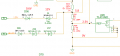# Who can explain how this over voltage detection circuit works?

#### ericgibbs

Joined Jan 29, 2010
16,800
hi cal,
Where is the circuit used?
Who designed it.?
E

#### ronsimpson

Joined Oct 7, 2019
2,535
U28, TL431 is the heart of the tooo complicated project. It is used where if "REF" is above 2.5V then pin-1 pulls low. If "REF" is below 2.5V then pin -1 is open.

U29 adds positive feedback. If U29 (& U38) are on, then R12 is shorted out and helps keep U28 on.

48VDC is divided down by to a voltage just under the 2.5V refence.

OV_TEST causes the 24V trip point to be lower.

12V gets subtracted by a Zener and fed into U28.

VR3 is a constant current source.

•calvin0908

#### calvin0908

Joined Mar 9, 2010
28
hi cal,
Where is the circuit used?
Who designed it.?
E
It only says it is an over voltage detection circuit, It was designed by a former colleague but he has gone

#### calvin0908

Joined Mar 9, 2010
28
hi cal,
Where is the circuit used?
Who designed it.?
E
Thanks Eric, your explanation gave me clue to dig more, it is very helpful.
You can see there is a 12V dc and a 30V dc parallel connected to R12, does this circuit can do over voltage detection for DC 12v & DC 30V also?
You said :" 12V gets subtracted by a Zener and fed into U28. "
If the Vz of BZX84C12 is 12.3V, Vr of BZX84C12 is 8V, what is the voltage level at the upper terminal of R12?

#### calvin0908

Joined Mar 9, 2010
28
U28, TL431 is the heart of the tooo complicated project. It is used where if "REF" is above 2.5V then pin-1 pulls low. If "REF" is below 2.5V then pin -1 is open.

U29 adds positive feedback. If U29 (& U38) are on, then R12 is shorted out and helps keep U28 on.

48VDC is divided down by to a voltage just under the 2.5V refence.

OV_TEST causes the 24V trip point to be lower.

12V gets subtracted by a Zener and fed into U28.

VR3 is a constant current source.
Thanks , your explanation gave me clue to dig more, it is very helpful.
You can see there is a 12V dc and a 30V dc parallel connected to R12, does this circuit can do over voltage detection for DC 12v & DC 30V also?
You said :" 12V gets subtracted by a Zener and fed into U28. "
If the Vz of BZX84C12 is 12.3V, Vr of BZX84C12 is 8V, what is the voltage level at the upper terminal of R12

#### calvin0908

Joined Mar 9, 2010
28
Thanks

#### calvin0908

Joined Mar 9, 2010
28
Welcome to join this discussion!

#### ronsimpson

Joined Oct 7, 2019
2,535
You said :" 12V gets subtracted by a Zener and fed into U28. "
The "12V" will loose 0.6V of D56 and about 12V from the Zener.
At or very neat the trip point, the voltage on the TL431 input will be 2.5V.
At the trip point, the voltage at the top of R12 2k will be 5.9V.
The "12V" will need to be above (0.6 + 12 + 5.8) for the TL431 to turn on and latch.
When the TL431 turns on, U29 shorts out the 2k resistor. For the TL431 to reset the 12V will need to be below (0.6 + 12 + 0.4 +2.5). Remember 0.6 if from D56, 12 is from Zener, 0.4 is the voltage across [U29//R2k], 2.5 is the voltage to turn on/off the TL431.#### calvin0908

Joined Mar 9, 2010
28
The "12V" will loose 0.6V of D56 and about 12V from the Zener.
At or very neat the trip point, the voltage on the TL431 input will be 2.5V.
At the trip point, the voltage at the top of R12 2k will be 5.9V.
The "12V" will need to be above (0.6 + 12 + 5.8) for the TL431 to turn on and latch.
When the TL431 turns on, U29 shorts out the 2k resistor. For the TL431 to reset the 12V will need to be below (0.6 + 12 + 0.4 +2.5). Remember 0.6 if from D56, 12 is from Zener, 0.4 is the voltage across [U29//R2k], 2.5 is the voltage to turn on/off the TL431.
View attachment 278585
Hi Ron,
Thanks for more details, I would like to summary your explanation as below: is it right?
If the 12V dc input voltage level >=0.6+12+5.8=18.4V, this voltage level will trigger the over voltage detection, the signal of OV_D (U38 is turned on ) will be high, right? then if the 12V dc input voltage level decreased to 0.6+12+2.5+0.4=15.5V, U38 will turned off, the signal of OV-D will be low, right?
please correct me if I am wrong.
Thanks
Calvin

•ronsimpson